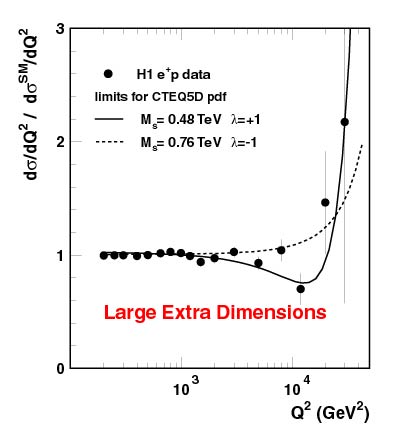# Search for Compositeness, Leptoquarks and Large Extra Dimensions in eq Contact Interactions at HERA

The H1 experiment has measured the cross section e+p-->e+X and compared to the neutral current expectations of the Standard Model of strong and electroweak interactions for momentum transfer Q2 ranging between 200 and 30000 GeV2 . At the highest Q2, where distance scales down to 1/1000 of the proton radius are probed, one could speculate that effects due to new substructures or new particles - should they exist - alter the basic interaction and therefore the measured cross section.

The data (see figure) show no significant deviation from the expectation of the Standard Model (SM) and are hence used to place exclusion limits. Deviations are conveniently expressed in so called contact interactions, which apart from a (heavy) mass scale depend on the chiral structure, i.e. the handedness of the eq-interaction. H1 was thus able to establish stringent lower limits on the mass scale in the range between 2 and 5 TeV for various chiral combinations of cross sections. Likewise the light quark radius is seen to be smaller than 1.7*10-16 cm so that deviations from the point like nature are severely restricted.A rather recent theoretical speculation allows for the exchange of virtual gravitons coupling to quarks and gluons propagating in (4+n) dimensions. If the number n of extra (compactified) dimensions is large (n>2) then effects might be visible in the H1 data. The observation of such effects could imply for gravity deviation from Newton's law in the sub-millimeter range. The lower limit that could be derived from the H1 data was set at a virtual graviton contribution of 0.48 TeV as can be seen from the figure that shows the ratio of the measurement and the SM expectation as a function of the momentum transfer Q2. The lines show the effect of putative gravitons interfering positively or negatively with the standard neutral current process.

Last Update Feb 15, 2000, E. Elsen